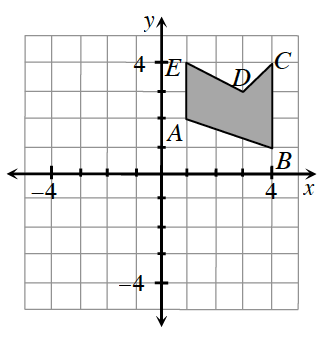### Home > INT2 > Chapter 9 > Lesson 9.3.1 > Problem9-80

9-80.Copy the graph at right onto graph paper.

1. If the shape $ABCDE$ were rotated about the origin $180^\circ$, where would point $A'$ be?

($-1, -2$)

1. If the shape $ABCDE$ were reflected across the $x$-axis, where would point $C'$ be?

A reflection across the $x$-axis means that the $y$-coordinates become negative.

2. If the shape $ABCD$ were translated so that each point ($x, y$) corresponds to ($x - 1, y + 3$), where would point $B'$ be?

In other words, subtract $1$ from the $x$-coordinate and add $3$ to the $y$-coordinate in point $B$.